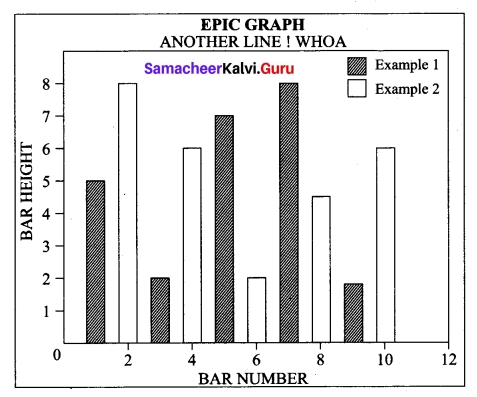# Tamil Nadu 12th Computer Science Model Question Paper 5 English Medium

Students can Download Tamil Nadu 12th Computer Science Model Question Paper 5 English Medium Pdf, Tamil Nadu 12th Computer Science Model Question Papers helps you to revise the complete Tamilnadu State Board New Syllabus and score more marks in your examinations.

## TN State Board 12th Computer Science Model Question Paper 5 English Medium

General Instructions:

1. The question paper comprises of four parts.
2. You are to attempt all the parts. An internal choice of questions is provided wherever applicable.
3. All questions of Part I, II, III and IV are to be attempted separately.
4. Question numbers 1 to 15 in Part I are Multiple Choice Questions of one mark each.
These are to be answered by choosing the most suitable answer from the given four alternatives and writing the option code and the corresponding answer
5. Question numbers 16 to 24 in Part II are two-mark questions. These are to be answered in about one or two sentences.
6. Question numbers 25 to 33 in Part III are three-mark questions. These are to be answered in about three to five short sentences.
7. Question numbers 34 to 38 in Part IV are five-mark questions. These are to be answered in detail Draw diagrams wherever necessary.

Time: 3 Hours
Maximum Marks: 70

PART – I

All questions are compulsory. [15 × 1 = 15]

Choose the most appropriate answer from the given four ‘alternatives and write the option code with the corresponding answer.

Question 1.
Bundling two values together into one can be considered as
(a) Pair
(b) Triplet
(c) Single
(a) Pair

Question 2.
The kind of scope of the variable ‘a’ used in the pseudo code given below
(i) Disp():
(ii) a: = 7
(iii) print a
(iv) Disp()
(a) Local
(b) Global
(c) Enclosed
(d) Built-in
(b) Global

Question 3.
Big Q is the reverse of…………
(a) Big O
(b) Big 0
(c) Big A
(d) Big S
(a) Big O

Question 4.
Extension of Python files is………..
(a) .Pyt
(b) .txt
(c) .Pdm
(d) .Py
(d) .PyQuestion 5.
The output of the Segment
for i in range (10, 0, 2)
print (i)
(a) 10 8 6 420
(b) 10 8 6 4 2
(c) 0 2 4 6 8 10
(d) Error
(d) Error

Question 6.
The bin() function returns a binary string prefixed with:
(a) 0
(b) 1
(c) 0b
(d) lb
(c) 0b

Question 7.
The positive and negative index value of’P’ in the string Strl = ‘COMPUTER’ are
(a) 3, -4
(b) 4, -4
(c) 3, -5
(d) 4, -5
(c) 3, -5

Question 8.
Which of the following set operation includes all the elements that are in two sets but not the one that are common to two sets?
(a) Symmetric difference
(b) Difference
(c) Intersection
(d) Union
(a) Symmetric difference

Question 9.
A variable prefixed with double underscore is
(a) private
(b) public
(c) protected
(d) static
(a) private

Question 10.
The data model developed by IBM is
(a) hierarchical
(b) relational
(c) network
(d) ER
(a) hierarchical

Question 11.
The SQL command to make a database as current active database is
(a) CURRENT
(b) USE
(c) DATABASE
(d) NEW
(b) USE

Question 12.
The expansion of CRLF is…………..
(a) Control Return and Line Feed
(b) Carriage Return and Form Feed
(c) Control Router and Line Feed
(d) Carriage Return and Line Feed
(d) Carriage Return and Line Feed

Question 13.
The function call statement of the segment………….
if_name_ == ‘_main_’:
main(sys.argv[1:])
is
(a) main(sys.argv[l:])
(b) _name_
(c) _main_
(d) argv
(b) _name_

Question 14.
Which is not a SQL clause?
(a) GROUP BY
(b) ORDER BY
(c) HAVING
(d) CONDITION
(d) CONDITIONQuestion 15.
To make a bar chart with Matplotlib, which function should be used?
(a) plt.bar()
(b) plt.chart()
(c) pip.bar()
(d) pip.chart()
(a) plt.bar()

PART – II

Answer any six questions. Question No. 21 is compulsory. [6 x 2 = 12]

Question 16.
What do you mean by Namespaces?
Namespaces are containers for mapping names of variables to objects.

Question 17.
What is searching? Write its types.
A search algorithm is the step-by-step procedure used to locate specific data among a collection of data. Types of searching algorithms are:

1. Linear search
2. Binary search
3. Hash search
4. Binary Tree search

Question 18.
Define Operator and Operand.
In computer programming languages operators are special symbols which represent computations, conditional matching etc. The value of an operator used is called operands. Operators are categorized as Arithmetic, Relational, Logical, Assignment etc. Value and variables when used with operator are known as operands.

Question 19.
What are the types of looping supported by Python?
Python provides two types of looping constructs:
while loop:
In the ‘while loop’, the condition is any valid Boolean expression returning True or False. The else part of while is optional part of while.

for loop:
‘for loop’ is the most comfortable loop. It is also an entry check loop. The condition is checked in the beginning and the body of the loop(statements-block 1) is executed if it is only True otherwise the loop is not executed.

Question 20.
What is the use of the operator += in python string operation?
Adding more strings at the end of an existing string is known as append (+=). The operator += is used to append a new string with an existing string.
Example:
>>> strl = “Welcome to”
>>> strl += “Leam Python”
>>> print (strl)
Welcome to Learn Python

Question 21.
What will be the output of the following snippet?
alpha = list(range(65, 70))
for x in alpha:
print(chr(x), end=’\t’)
Output:
A B C D EQuestion 22.
What is the use of WHERE clause in SQL?
The WHERE clause in the SELECT command specifies the criteria for getting the desired result. The general form of SELECT command with WHERE Clause is:
SELECT[,,….] FROM WHERE condition>;
The relational operators like =, <, <=, >, >=, <> can be used to compare two values in the SELECT command used with WHERE clause. The logical operaors OR, AND and NOT can also be used to connect search conditions in the WHERE clause. For example:
SELECT Admno, Name, Age, Place FROM Student WHERE (Age>=18 AND Place = “Delhi”);

Question 23.
What are the steps involved in file operation of Python?
When you want to read from or write to a file ,you need to open it. Once the reading is over it needs to be closed. So that, resources that are tied with the file are freed. Hence, in Python, a file operation takes place in the following order.
Step 1 → Open a file
Step 2 → Perform Read or write operation
Step 3 → Close the file

Question 24.
Distinguish compiler and interpreter.

 Compiler Interpreter 1. It converts the whole program at a time line by line execution of the source code 2. It is faster It is slow 3. Error detection is difficult, e.g., C++ It is easy. e.g., Python

PART – III

Answer any six questions. Question No. 29 is compulsory. [6 x 3 = 18]

Question 25.
Why strlen is called pure function?
strlen (s) is called each time and strlen needs to iterate over the whole of ‘s’. If the compiler is smart enough to work out that strlen is a pure function and that ‘s’ is not updated in the loop, then it can remove the redundant extra calls to strlen and make the loop to execute only one time. This function reads external memory but does not change it, and the value returned derives from the external memory accessed.

Question 26.
Which strategy is used for program designing? Define the strategy.
We are using here a powerful strategy for designing programs: ‘wishful thinking’.
Wishful Thinking is the formation of beliefs and making decisions according to what might be pleasing to imagine instead of by appealing to reality.

Question 27.
Which jump statement is used as placeholder? Why?
pass statement is generally used as a placeholder. When we have a loop or function that is to be implemented in the future and not now, we cannot develop such functions or loops with empty body segment because the interpreter would raise an error. So, to avoid this we can use pass statement to construct a body that does nothing.

Question 28.
What are the points to be noted while defining a function?

1. Function blocks begin with the keyword “def ” followed by function name and parenthesis ().
2. Any input parameters or arguments should be placed within these parentheses when you define a function.
3. The code block always comes after a colon (:) and is indented.
4. The statement “return [expression]” exits a function, optionally passing back an expression to the caller. A “return” with no arguments is the same as return None.Question 29.
Write a Python program to display the given pattern
COMPUTER
COMPUTE
COM PUT
COMPU
COMP
COM
CO
C
Program:
strl = “COMPUTER”
index = len(strl)
for i in strl:
print(strl[: index])
index – = 1

Question 30.
What is the output of the following program?
class Greeting:
def_init_(self, name):
self._name = name
def displayf self):
print (“Good Morning”, self._name)
obj.displayO

Question 31.
Explain Cartesian product with a suitable example.
PRODUCT OR CARTESIAN PRODUCT (Symbol: X)
Cross -product is a way of combining two relations. The resulting relation contains, both relations being combined.
A × B means A times B, where the relation A and B have different attributes.
This type of operation is helpful to merge columns from two relations.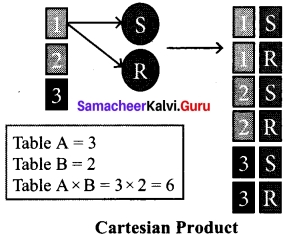Question 32.
Write a short note on (i) fetchallf) (ii) fetchonef) (iii) fetchmany
cursor.fetchall() -fetchall ()method is to fetch all rows from the database table.
cursor. fetchoneQ – The fetchone () method returns the next row of a query result set or None in case there is no row left.
cursor, fetchmany() method that returns the next number of rows (n) of the result set

Question 33.
Write a Python code to display the following chart.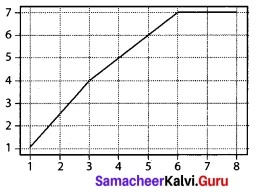Import matplotlib.pyplot as pit
x = [1, 2, 3,4, 5, 7,8]
y = [1,2.5, 4, 5, 6, 7, 7]
plt.plot([1, 2, 3, 4])
plt.show()

PART – IV

Answer all the following questions. [5 x 5 = 25]

Question 34 (a).
Write any five benefits in using modular programming.
The benefits of using modular programming include

1. Less code to be written.
2. A single procedure can be developed for reuse, eliminating the need to retype the code many times.
3. Programs can be designed more easily because a small team deals with only a small part of the entire code.
4. Modular programming allows many programmers to collaborate on the same application.
5. The code is stored across multiple files.
6. Code is short, simple and easy to understand.
7. Errors can easily be identified, as they are localized to a subroutine or function.
8. The same code can be used in many applications.
9. The scoping of variables can easily be controlled.(OR)

(b) Explain input() and print() functions of Python with example.
Input() function:
In Python, input() function is used to accept data as input at run time. The syntax for input() function is,
Variable = input (“prompt string”)
Where, prompt string in the syntax is a statement or message to the user, to know what input can be given.
If a prompt string is used, it is displayed on the monitor; the user can provide expected data from the input device. The input() takes whatever is typed from the keyboard and stores the entered data in the given variable. If prompt string is not given in input() no message is displayed on the screen, thus, the user will not know what is to be typed as input.
Example 1:
input() with prompt string
>>> city = input (“Enter Your City: ”)
>>> print (“I am from“, city)

Example 2:
input() without prompt string
>>> city = input()
Rajarajan
>>> print (I am from”, city)
I am from Rajarajan

The print() function:
In Python, the print() function is used to display result on the screen. The syntax for print() is as follows:
Example:
print (“string to be displayed as output ”)
print (variable)
print (“String to be displayed as output ”, variable)
print (“String 1 ”, variable, “String 2”, variable, “String 3”………. )

Example:
>>> print (“Welcome to Python Programming”)
Welcome to Python Programming
>>> x = 5
>>> y = 6
>>> z = x + y
>>> print (z)
11
>>> print (“The sum = ”, z)
The sum = 11
>>> print (“The sum of ”, x, “ and ”, y, “ is ”, z)
The sum of 5 and 6 is 11

The print () evaluates the expression before printing it on the monitor. The print () displays an entire statement which is specified within print (). Comma (,) is used as a separator in print () to print more than one item.

Question 35 (a).
Write a detail note on for loop in Python.
for loop
for loop is the most comfortable loop. It is also an entry check loop. The condition is checked in the beginning and the body of the loop(statements-block 1) is executed if it is only True otherwise the loop is not executed.
Syntax:
for counter_variable in sequence:
statements block 1
# optional block
[else:
statements block 2]
The counter_variable mentioned in the syntax is similar to the control variable that we used in the for loop of C++ and the sequence refers to the initial, final and increment value. Usually in Python, for loop uses the range() function in the sequence to specify the initial, final and increment values. range() generates a list of values starting from start till stop-1.

The syntax of range() is as follows:
range (start, stop, [step])
Where,
start – refers to the initial value
stop – refers to the final value
step – refers to increment value, this is optional part.

Examples for range()
range (1, 30, 1) will start the range of values from 1 and end at 29
range (2, 30, 2) will.start the range of values from 2 and end at 28
range (30, 3, -3) will start the range of values from 30 and end at 6
range (20) will consider this value 20 as the end value(or upper limit) and starts the range count from 0 to 19 (remember always range() will work till stop -1 value only)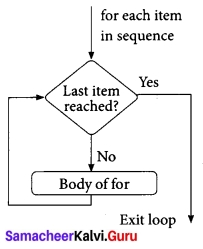#program to illustrate the use of for loop – to print single digit even number
for i in range (2, 10, 2):
print (i, end = ‘ ‘)
Output:
2 4 6 8[OR]

(b) Explain the different types of functions in Python with example.
Types of Functions
Basically, we can divide functions into the following types:

1. User-defined Functions
2. Built-in Functions
3. Lambda Functions
4. Recursion Functions
 Functions Description User-defined functions Functions defined by the users themselves. Built-in functions Functions that are inbuilt with in Python. Lambda functions Functions that are anonymous un-named function. Recursion functions Functions that calls itself is known as recursive.

1. Syntax for User defined function
def < function_name ([parameter 1, parameter2 …… ])> :

return
Example:
def hello():
print (“hello – Python”)
return

1. Functions help us to divide a program into modules. This makes the code easier to manage.
2. It implements code reuse. Every time you need to execute a sequence of statements, all you need to do is to call the function.
3. Functions, allows us to change functionality easily, and different programmers can work on different functions.

2. Anonymous Functions
In Python, anonymous function is a function that is defined without a name. While normal functions are defined using the def keyword, in Python anonymous functions are defined using the lambda keyword. Hence, anonymous functions are also called as lambda functions.
The use of lambda or anonymous function:

1. Lambda function is mostly used for creating small and one-time anonymous function.
2. Lambda functions are mainly used in combination with the functions like filter(), map() and reduce().

Syntax of Anonymous Functions:
The syntax for anonymous functions is as follows:
lambda [argument(s)]:expression
Example:
sum = lambda argl, arg2: argl + arg2
print (‘The Sum is :’, sum(30,40))
print (‘The Sum is :’, sum(-30,40))
Output:
The Sum is : 70
The Sum is : 10
The above lambda function that adds argument argl with argument arg2 and stores the result in the variable sum. The result is displayed using the print().

3. Functions using libraries
Built-in and Mathematical functions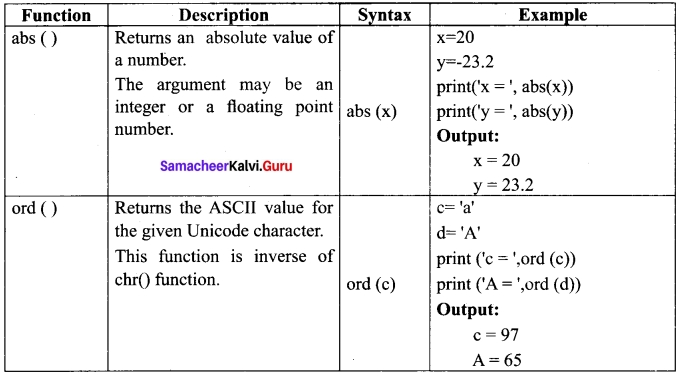4. Recursive functions
When a function calls itself is known as recursion. Recursion works like loop but sometimes it makes more sense to use recursion than loop. You can convert any loop to recursion.
Example:
def fact(n):
if n = = 0:
return 1
else:
return n * fact (n – 1)
print (fact (0))
print (fact (5))
Output:
1
120Question 36 (a).
Explain about the find() function in Python with example.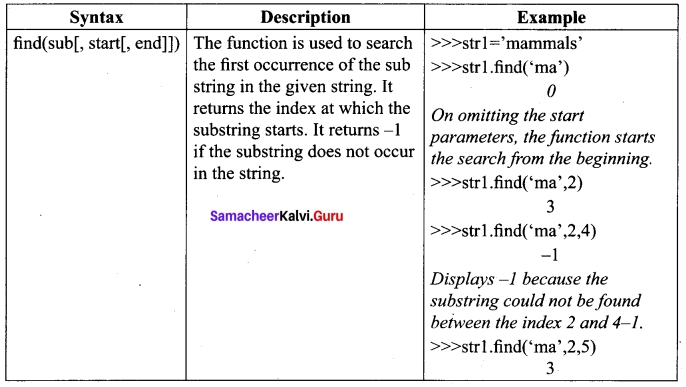(OR)

(b) Compare remove(), pop() and clear() function in Python.
The remove( ) function can also be used to delete one or more elements if the index value is not known. Apart from remove() function, pop() function can also be used to delete an element using the given index value. pop() function deletes and returns the last element of a list if the index is not given.
The function clear() is used to delete all the elements in list, it deletes only the elements and retains the list. Remember that, the del statement deletes entire list.
Syntax:
List.remove(element)
# to delete a particular element
List.pop(index of an element)
List, clear ()
Example:
>>> MyList = [12, 89, 34,’Kannan’, ‘Gowrisankar’, ‘Lenin’]
>>> print(MyList)
[12, 89, 34, ‘Kannan’, ‘Gowrisankar’, ‘Lenin’]
>>> MyList.remove(89)
>>> print(MyList)
[12, 34, ‘Kannan’, ‘Gowrisankar’, ‘Lenin’]
In the above example, MyList has been created with three integer and three string elements, the following print statement shows all the elements available in the list. In the statement.
>>> MyList.remove(89), deletes the element 89 from the list and the print statement shows the remaining elements.
Example:
>>> MyList.pop(l)
34
>>> print(MyList)
[12, ‘Kannan’, ‘Gowrisankar’, ‘Lenin’]
In the above code, pop() function is used to delete a particular element using its index value, as soon as the element is deleted, the pop() function shows the element which is deleted. pop() function is used to delete only one element from a list. Remember that, del statement deletes multiple elements.
Example:
>>> MyList.clear()
>>> print(MyList)
[]
In the above code, clear() function removes only the elements and retains the list. When you try to print the list which is already cleared, an empty square bracket is displayed without any elements, which means the list is empty.Question 37 (a).
Explain the components of DBMS.
Components of DBMS: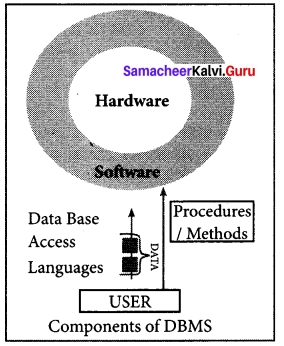The Database Management System can be divided into five major components as follows:

1. Hardware
2. Software
3. Data
4. Procedures/Methods
5. Database Access Languages

1. Hardware: The computer, hard disk, I/O channels for data, and any other physical component involved in storage of data.
2. Software: This main component is a program that controls everything. The DBMS software is capable of understanding the Database Access Languages and interprets into database commands for execution.
3. Data: It is that resource for which DBMS is designed. DBMS creation is to store and utilize data.
4. Procedures/Methods: They are general instructions to use a database management system such as installation of DBMS, manage databases to take backups, report generation, etc.
5. DataBase Access Languages: They are the languages used to write commands to access, insert, update and delete data stored in any database.

[OR]

(b) What are the components of SOL? Write the commands in each.
Components of SQL
SQL commands are divided into five categories: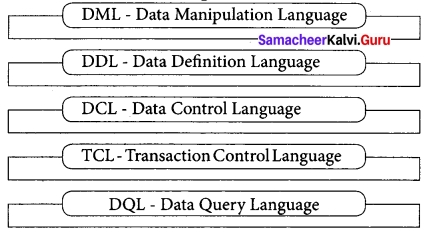a. Data Definition Language
The Data Definition Language (DDL) consist of SQL statements used to define the database structure or schema. It simply deals with descriptions of the database schema and is used to create and modify the structure of database objects in databases.
SQL commands which comes under Data Definition Language are:

 Create To create tables in the database. Alter Alters the structure of the database. Drop Delete tables from database. Truncate Remove all records from a table, also release the space occupied by those records.

b. Data Manipulation Language
A Data Manipulation Language (DML) is a computer programming language used for adding (inserting), removing (deleting), and modifying (updating) data in a database.
SQL commands which comes under Data Manipulation Language are :

 Insert Inserts data into a table Update Updates the existing data within a table. Delete Deletes all records from a table, but not the space occupied by them.

c. Data Control Language:
A Data Control Language (DCL) is used for controlling privileges in the database SQL commands: GRANT, REVOKE

d. Transactional Control Language;
Transactional control language (TCL) is used to manage transactions i.e. changes made to the data in the database.
SQL commands: COMMIT, ROLLBACK, SAVEPOINT.

e. Data Query Language
The Data Query Language (DQL) have commands to query or retrieve data from the database. SQL commands: SELECT.Question 38 (a).
Explain the following operators in Relational Algebra with suitable example
1. Union, (∪) 20 Intersection (∩)
UNION (Symbol :∪)
It includes all tuples that are in tables A or in B. It also eliminates duplicates. Set A Union Set B would be expressed as A ∪ B
Example 2
Consider the following tables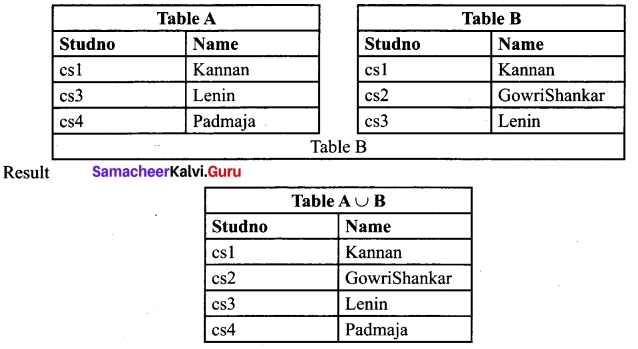INTERSECTION (symbol: ∩) A ∩ B
Defines a relation consisting of a set of all tuple that are in both in A and B. However, A and B must be union-compatible.
Example 5 (using Table B)

 Table A ∩ B Studno Name csl Kannan cs3 Lenin

[OR]

(b) Draw the output for the following data visualization plot.
import matplotlib.pyplot as pit
plt.bar([1, 3, 5, 7, 9],[5, 2, 7, 8, 2], label=”Example one”)
plt.bar([2, 4, 6, 8, 10],[8, 6, 2, 5, 6], label=”Example two”, color=’g’)
plt.legendO
plt.xlabel(‘bar number’)
plt.ylabel(‘bar height’)
plt.title(‘Epic Graph\nAnother Line! Whoa’)
plt.show()